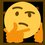# Power Rule

\begin{aligned} \big({ x }^{ n }\big)\prime & = \lim _{ h\rightarrow 0 }{ \frac { { (x+h) }^{ n }-\big({ x }^{ n }\big) }{ h } } \\ \quad & = \lim _{ h\rightarrow 0 }{ \frac { \bigg(\displaystyle \sum _{ k=0 }^{ n }{ \binom{n}{k} { x }^{ n-k }{ h }^{ k } } \bigg)-{ x }^{ n } }{ h } } \\ \quad & = \lim _{ h\rightarrow 0 }{ \frac { { x }^{ n }+\bigg(\displaystyle \sum _{ k=1 }^{ n }{ \binom{n}{k} { x }^{ n-k }{ h }^{ k } } \bigg)-{ x }^{ n } }{ h } } \\ \quad & = \lim _{ h\rightarrow 0 }{ \frac \sum _{ k=1 }^{ n }{ h\binom{n}{k} { x }^{ n-k }{ h }^{ k-1 } } }{ h } } \\ \quad & = \lim _{ h\rightarrow 0 }{ \frac { h\bigg(\displaystyle \sum _{ k=1 }^{ n }{ \binom{n}{k} { x }^{ n-k }{ h }^{ k-1 } } \bigg) }{ h } } \\ \quad & = \lim _{ h\rightarrow 0 }{ \sum _{ k=1 }^{ n }{ \binom{n}{k} { x }^{ n-k }{ h }^{ k-1 } } } \\ \quad & = \lim _{ h\rightarrow 0 }{ \bigg(\binom{n}{1} { x }^{ n-1 }{ h }^{ 0 }+\binom{n}{2} { x }^{ n-2 }{ h }^{ 1 }+\binom{n}{3} { x }^{ n-3 }{ h }^{ 2 }+\cdots \bigg) } \\ \quad & = \lim _{ h\rightarrow 0 }{ \Bigg(n{ x }^{ n-1 }+h\bigg(\binom{n}{2} { x }^{ n-2 }+\binom{n}{3} { x }^{ n-3 }{ h }^{ 1 }+\cdots \bigg)\Bigg) } \\ \quad & = n{ x }^{ n-1 }+0\bigg(\binom{n}{2} { x }^{ n-2 }+\binom{n}{3} { x }^{ n-3 }{ 0 }^{ 1 }+\cdots \bigg) \\ \quad & = \boxed { n{ x }^{ n-1 } } \end{alignedNote by Gandoff Tan
2 years, 1 month ago

This discussion board is a place to discuss our Daily Challenges and the math and science related to those challenges. Explanations are more than just a solution — they should explain the steps and thinking strategies that you used to obtain the solution. Comments should further the discussion of math and science.

When posting on Brilliant:

• Use the emojis to react to an explanation, whether you're congratulating a job well done , or just really confused .
• Ask specific questions about the challenge or the steps in somebody's explanation. Well-posed questions can add a lot to the discussion, but posting "I don't understand!" doesn't help anyone.
• Try to contribute something new to the discussion, whether it is an extension, generalization or other idea related to the challenge.

MarkdownAppears as
*italics* or _italics_ italics
**bold** or __bold__ bold
- bulleted- list
• bulleted
• list
1. numbered2. list
1. numbered
2. list
Note: you must add a full line of space before and after lists for them to show up correctly
paragraph 1paragraph 2

paragraph 1

paragraph 2

[example link](https://brilliant.org)example link
> This is a quote
This is a quote
    # I indented these lines
# 4 spaces, and now they show
# up as a code block.

print "hello world"
# I indented these lines
# 4 spaces, and now they show
# up as a code block.

print "hello world"
MathAppears as
Remember to wrap math in $$ ... $$ or $ ... $ to ensure proper formatting.
2 \times 3 $2 \times 3$
2^{34} $2^{34}$
a_{i-1} $a_{i-1}$
\frac{2}{3} $\frac{2}{3}$
\sqrt{2} $\sqrt{2}$
\sum_{i=1}^3 $\sum_{i=1}^3$
\sin \theta $\sin \theta$
\boxed{123} $\boxed{123}$

Sort by:

I see an error in the fifth line of your reasoning to the power rule. Since you are summing the h's over and over again you can simply factor it out so once it is factored out the h no longer appears in the summand.

- 2 years ago

Oops. Thanks for pointing out.

- 2 years ago

No Problem. I have one more question, do you like the Joy Of Problem solving course. I seem to love it. I want to know from you if there are other problem solving courses too on this. Thanks.

- 2 years ago

I like it. I think you can try the 'Ace the AMC' course.

- 2 years ago

I wonder if there is any way to show this without calculus? @Gandoff Tan

- 1 year, 2 months ago

@Brilliant Mathematics, @Creative Biogene is not talking about mathematics.

- 1 year, 2 months ago

Thank you again! We will take action on this account.

Staff - 1 year, 2 months ago